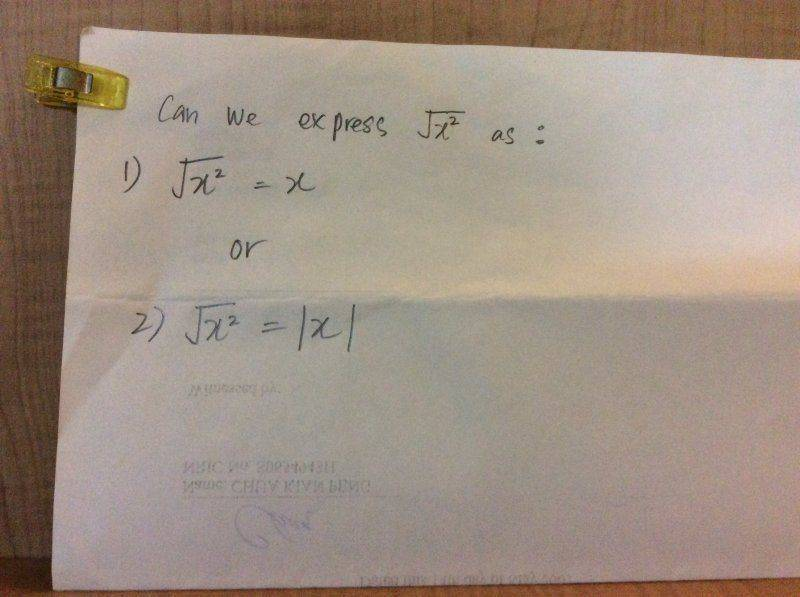# Basic algebra question

LiHJ
<< Mentor Note -- OP has been advised to type their questions into the forum next time, instead of inserting images >>

1. Homework Statement

Dear Mentors and PF helpers,

I have a question from today's lesson.## The Attempt at a Solution

Is 1) and 2) both acceptable? If only of of them is acceptable, is there any reason why?

Thanks for your time

Last edited by a moderator:

## Answers and Replies

Homework Helper
Only 2.
The ## \sqrt{} ## implies that it only returns the positive square root of a number. That is why you will often see ##\pm \sqrt{} ## in formulas.

Homework Helper
For x any real number, $\sqrt{x^2}= |x|$, NOT $\sqrt{x}= x$

That is easily seen by taking x to be any negative number, say x= -2. In that case, $x^2= (-2)^2= 4$ and $\sqrt{x^2}= \sqrt{4}= 2$, NOT -2.

Last edited by a moderator:
Homework Helper
However, if you know something about x, like it is positive, then you may use the notation in (1) .
Also, if you have ##y = x^2##, then ##x = \pm \sqrt{y}##.